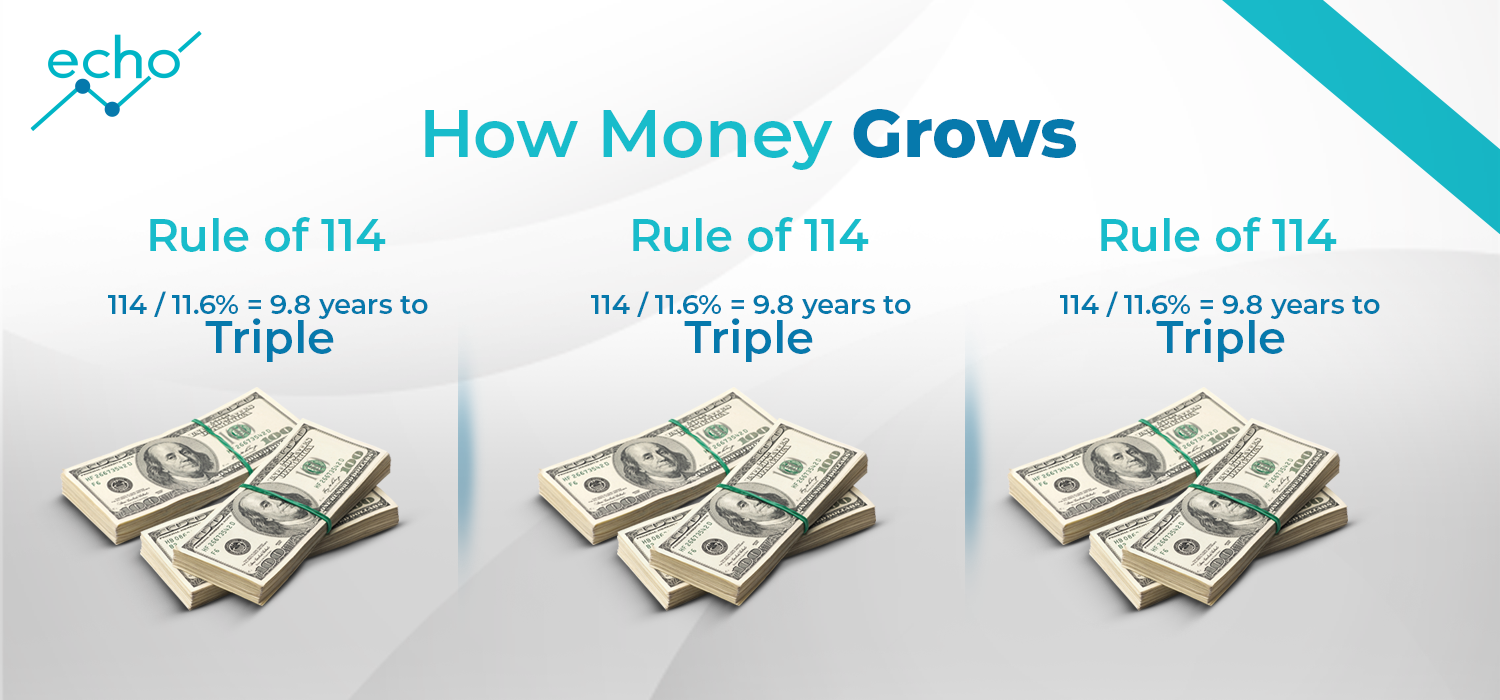# Rule of 114

Some of you might have heard of "Rule of 72" which simply states that money invested in an account bearing interest r, will double its principal in 72/r years when compounded annually. If you invest a \$1 at 3% interest compounded annually, you will end up with \$2 in approximately 72/3=24 years.

So , what is Rule of 114? Well, what if we want to know the time it takes to triple our initial principal given an interest rate, r. Just as you guess it, to triple our initial investment at an interest rate of 3% compounded annually, it will take approximately 114/3= 38 years. Lets see how we obtain the number 114 using the same reasoning for getting 72 in the rule of 72.